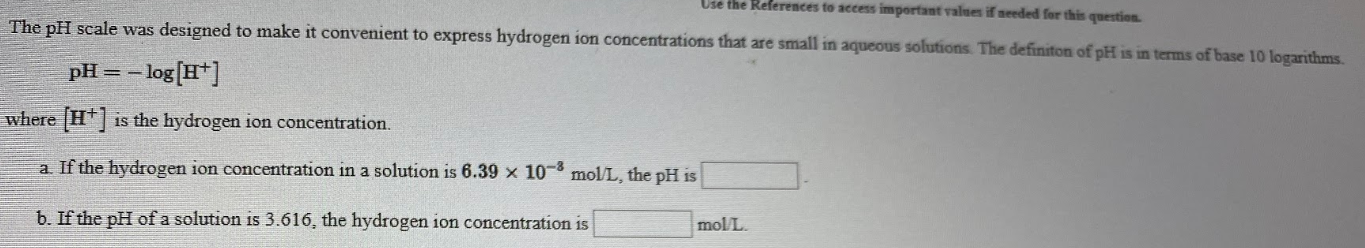# The pH scale was designed to make it convenient to express hydrogen ion concentration that are small in aqueous solutions The definition of pH is in terms of base 10 logarithms. pH = -log[H^+] where [H^+] is the hydrogen ion concentration. a) If the hydrogen ion concentration in a solution is 6.39 x 10^-8 mol/L, the pH is_____. b) If the pH of a solution is 3.616, the hydrogen ion concentration is _____ mol/L.